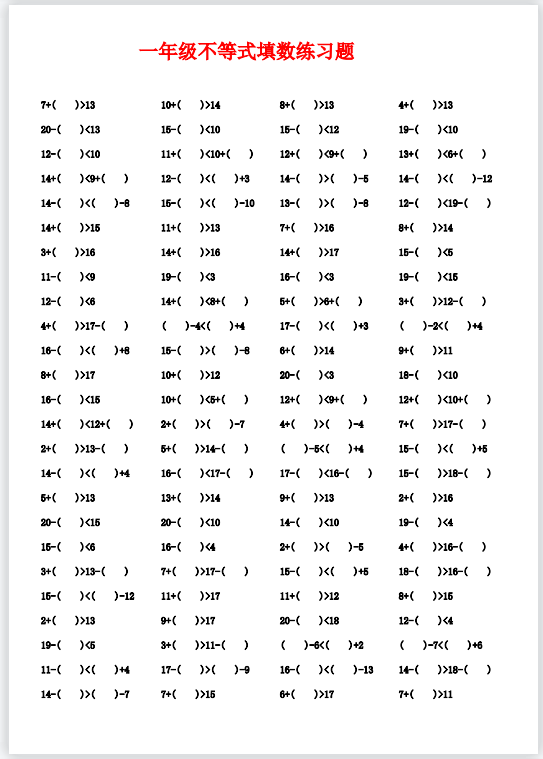7+( )>13 10+( )>14 8+( )>13 4+( )>13 20-( )<13 15-( )<10

15-( )<12 19-( )<10 12-( )<10 11+( )<10+( )  12+( )<9+( )  13+( )<6+( )

14+( )<9+( )  12-( )<( )+3  14-( )>( )-5  14-( )<( )-12  14-( )<( )-8  15-( )<( )-10

13-( )>( )-8  12-( )<19-( )